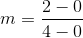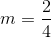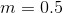## Example Questions

### Example Question #1 : How To Find The Slope Of A Tangent Line

There is a circle on a coordinate plain. Its perimeter passes through the point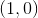. At this point meets a tangent line, which also passes through the point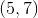. What is the slope of the line perpindicular to this tangent line?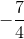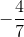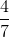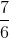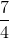Explanation:

In this kind of problem, it's important to keep track of information given about your line of interest. In this case, the coordinates given set up the stage for us to be able to get to our line of focus - the line perpendicular to the tangent line. In order to determine the perpendicular line's slope, the tangent line's slope must be calculated. Keeping in mind that: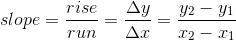where y2,x2 and y1,x1 are assigned arbitrarily as long as the order of assignment is maintained.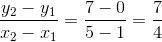which is the slope of the tangent line.

To calculate the perpendicular line, we have to remember that the product of the tangent slope and the perpendicular slope will equal -1.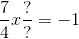, the perpendicular slope can then be calculated as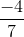### Example Question #2 : How To Find The Slope Of A Tangent Line

Find the slope of the tangent line to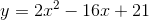where.Explanation:

To find the slope of the tangent line, we must take the derivative.

By using the Power Rule we will be able to find the derivative: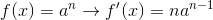Therefore derivative of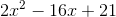is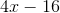.

Now we plug in, giving us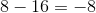.

### Example Question #3 : How To Find The Slope Of A Tangent Line

A line runs tangent to a circle at the point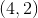. The line runs through the origin. Find the slope of the tangent line.

Cannot be determined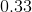Explanation:

The only two bits of information that are given for the tangent line is that it runs through the pointsand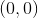. With these two points, the line's slope can be easily calculated through the equation: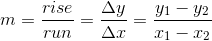whereis slope,is the-coordinate of the points, andis the-coordinates of the points.

Slope can be calculated through substituting in for the given values: